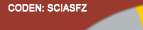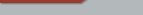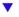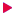| Home  | About ScienceAsia  | Publication charge  | Advertise with us  | Subscription for printed version  | Contact usEditorial Board Journal Policy Instructions for Authors Online submission Author Login Reviewer Login Volume 46 Number 2 Volume 46 Number 1 Volume 46S Number 1 Volume 45 Number 6 Volume 45 Number 5 Volume 45 Number 4 Earlier issuesVolume 40 Number 5Volume 40 Number 6 Volume 41 Number 1previous article next article1

Research articles

ScienceAsia 40 (2014): 451-455 |doi: 10.2306/scienceasia1513-1874.2014.40.451

# On the solutions and conservation laws for the Sharma-Tasso-Olver equation

## Andrew Gratien Johnpillaia, Chaudry Masood Khaliqueb,*

ABSTRACT:     We study the Sharma-Tasso-Olver equation from the Lie symmetry point of view. We derive the Lie point symmetry generators of the equation and classify them to obtain the optimal system of one-dimensional subalgebras of the Lie symmetry algebra of the equation. These subalgebras are then used to construct symmetry reductions for the equation. We obtain the general solution of the nonlinear second-order ordinary differential equation which results from the symmetry reduction for the travelling wave group-invariant solutions of the equation by transforming it into a linear third-order ordinary differential equation through a Riccati transformation. Then we show that one can easily obtain the travelling wave exact group-invariant solutions for the underlying equation by using the general solution of the linearized third-order ordinary differential equation and the Riccati transformation. We also construct conservation laws for the underlying equation by making use of the multiplier method.